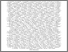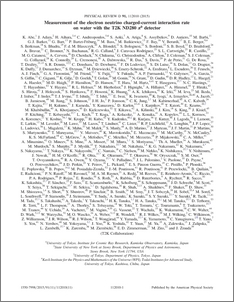# Measurement of the electron neutrino charged-current interaction rate on water with the T2K ND280 pi-zero detector

, T2K Collaboration (2015) Measurement of the electron neutrino charged-current interaction rate on water with the T2K ND280 pi-zero detector. Physical Review D, 91 (11). ISSN 1550-7998

Other (pdf)
1503.08815v4 - Accepted VersionPreview
PDF (PhysRevD.91.112010)
PhysRevD.91.112010.pdf - Published Version

## Abstract

This paper presents a measurement of the charged current interaction rate of the electron neutrino beam component of the beam above $1.5$~GeV using the large fiducial mass of the T2K $\pi^0$ detector. The predominant poriton of the $\nu_e$ flux ($\sim$85 %) at these energies comes from kaon decays. The measured ratio of the observed beam interaction rate to the predicted rate in the detector with water targets filled is 0.89 $\pm$ 0.08 (stat.) $\pm$ 0.11 (sys.), and with the water targets emptied is 0.90 $\pm$ 0.09 (stat.) $\pm$ 0.13 (sys.). The ratio obtained for the interactions on water only from an event subtraction method is 0.87 $\pm$ 0.33 (stat.) $\pm$ 0.21 (sys.). This is the first measurement of the interaction rate of electron neutrinos on water, which is particularly of interest to experiments with water Cherenkov detectors.

Item Type:
Journal Article
Journal or Publication Title:
Physical Review D